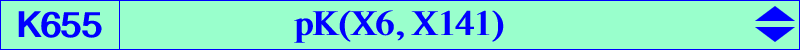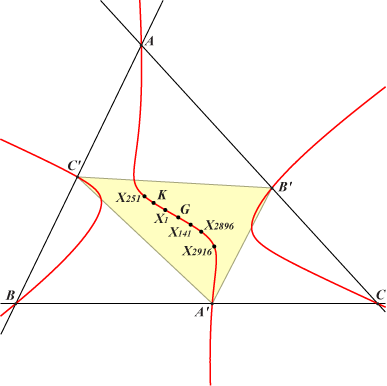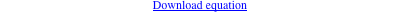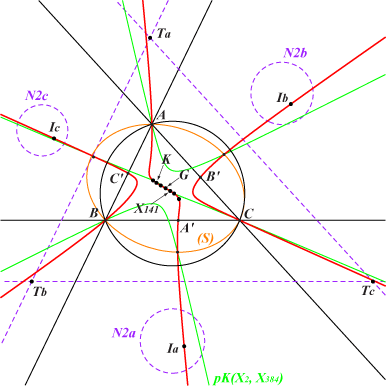∑ (b^2 + c^2) x (c^2 y^2 - b^2 z^2) = 0X(1), X(2), X(6), X(141), X(251), X(2896), X(2916), X(3961), X(7194) excenters A', B', C' : cevians of X(141) in ABC common points of the Steiner ellipse (S) and pK(X2, X384) cevians of X(251) in A'B'C' hence K655 is a pK with pivot X(251) in A'B'C'The radical axes of the circumcircle (O) and the second Neuberg circles N2a, N2b, N2c bound a triangle TaTbTc homothetic to ABC at X(251) and to the medial triangle at the barycentric product X(141) x X(7829) with SEARCH = 3.21492390998425. TaTbTc is perspective to the cevian triangle of every point on the cubic K655. TaTbTc is orthologic to the cevian triangle of every point on K007. *** The isotomic transform of K655 is pK(X76, X8024). The symbolic substitution SS{a -> √a} transforms K655 into K328. K655 is a member of the Thomson-Grebe pencil. See Table 13.